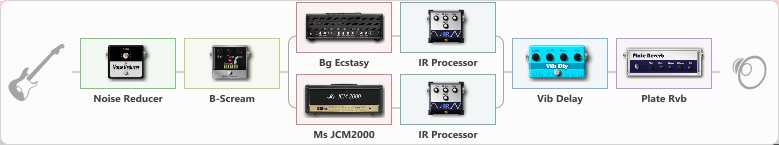# Bog JCM mix Truss

Discussion in 'ToneLib-GFX presets' started by truss van halen, Mar 7, 2022.

1. ### truss van halenWell-Known Member

Bog JCM mix Truss

Preset name: Carlinoss

Effects chain:Effect: "Noise Reducer" (Dynamics / Filter), active - "yes"
{
"Sens" = 100
"Mode" = Hard
}

Effect: "B-Scream" (Overdrive / Distortion), active - "yes"
{
"Drive" = 78
"Tone" = 28
"Level" = 65
}

Effect: "Splitter" (Dynamics / Filter)
{
"A-Bypass" = Off
"A-Pan" = -41
"A-Level" = 55
"B-Bypass" = Off
"B-Pan" = 40
"B-Level" = 55
"Width" = 0

'A' branch:
{

Effect: "Bg Ecstasy" (Amp simulators), active - "yes"
{
"Gain" = 100
"Bass" = 59
"Middle" = 59
"Treble" = 41
"Presence" = 56
"Master" = 73
"Level (dB)" = 5
}

Effect: "IR Processor" (Cabinets), active - "yes"
{
"IR" = archip_dc
"Low Cut (Hz)" = 0
"Hi Cut (kHz)" = 10.5
"Mix" = 100
"Level (dB)" = 2
}
}
'B' branch:
{

Effect: "Ms JCM2000" (Amp simulators), active - "yes"
{
"Gain" = 50
"Bass" = 74
"Middle" = 48
"Treble" = 50
"Presence" = 45
"Master" = 72
"Level (dB)" = 7
}

Effect: "IR Processor" (Cabinets), active - "yes"
{
"IR" = charv.1_dc
"Low Cut (Hz)" = 0
"Hi Cut (kHz)" = 20.0
"Mix" = 100
"Level (dB)" = 1
}
}
}

Effect: "Vib Delay" (Modulation / Sfx), active - "yes"
{
"Speed" = 4.2
"Depth" = 38
"Feedback" = 44
"Time" = 6.9
"Mix" = 32
"Mode" = 0
}

Effect: "Plate Rvb" (Reverberation), active - "yes"
{
"Time" = 4.3
"PreDelay" = 30
"LoDamp" = 17
"HiDamp" = 25
"Mix" = 42
}

Note: You will need to download and install the ToneLib-GFX software to use the preset.

#### Attached Files:

• ###### Bog_JCM_mix_Truss.tlgfx
File size:
61.5 KB
Views:
2,058
NikStar and Kormier like this.